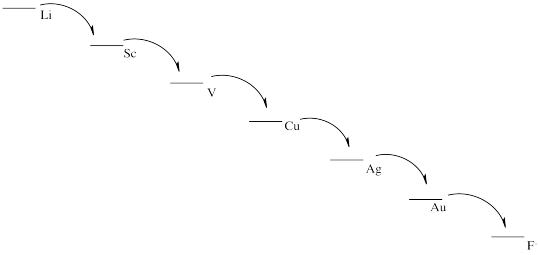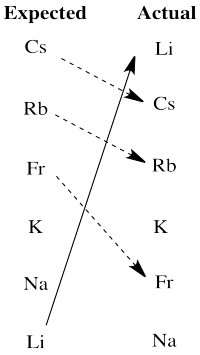# RO13. Solutions for Selected Problems

### Problem RO1.1.

a) Ag+ b) Ni2+ c) Mn7+ d) Cr6+ e) Cu3+ f) Fe3+ g)Os8+ h) Re5+

### Problem RO1.3.

a) Pb2+ & S2- b) Sn4+ & 2 O2- c) Hg2+ & S2- d) Fe4+ & 2 S2- e) 2 Fe3+ & 3 O2- f) 2 Fe3+ & 1 Fe2+ & 4 O2-

### Problem RO1.4.

Probably Fe2+, to replace Zn2+ ions.

### Problem RO1.5.

a) C 2+ b) C4+ c) 4- d) C0 e) C3+

### Problem RO1.7.

a) Mg2+ b) Cu2+ c) Mn3+ d) Ca2+ e) Mn2+ f) Mn2+

### Problem RO1.8.

a) Cu(II), Fe(II) b) Zn(II), Fe(III) c) Be(II), Al(III) d) Cu(I), Fe(III) e) Cu(II), Al(III)

### Problem RO2.1.

1. Cu → Cu(+ + e-
2. Fe3+ + 3 e-→ Fe
3. Mn → Mn3+ + 3 e-
4. Zn2+ + 2 e- → Zn
5. 2 F- → F2 + 2 e-
6. H2 → 2 H+ + 2 e-

### Problem RO2.2.

1. Cu(I) + Fe(III) → Cu(II) + Fe(II)
2. Cu(I) + Ag(0) → Cu(0) + Ag(I)
3. 3 F2 + 2 Fe → 6 F- + 2 Fe(III)
4. 2 Mo3+ + 3 Mn → 2 Mo + 3 Mn2+

### Problem RO2.3.

1. MnO2 + 2 H+ + 2 e- → Mn(OH)2
2. 2 NO + 2 e- + 2 H+ → N2O + H2O
3. HPO32- + 2 e- + 3 H+ → H2PO2- + H2O
4. Sn(OH)62- + 2 e- + 3 H+ → HSnO2- + 4 H2O

### Problem RO3.1.

MnO4- : 4 x O2- (= 8-) + Mn7+ = 1- overall

MnO2 : 2 x O2- (= 4-) + Mn4+ = neutral overall

difference = 3 e-

### Problem RO3.2.

a) SO42- : 4 x O2- (= 8-) + S6+ = 2- overall

S2O82- : 8 x O2- (= 16-) + 2 x S7+ (= 14+) = 2- overall

difference = 1 e- per S, or 2 e- overall

S2O82- + 2 e- → 2 SO42-

b) HPO32- : 3 x O2- (= 6-) + H+ + P3+ = 2- overall

P : P(0)

difference = 3 e-

HPO32- + 3 e- + 5 H+ → P + 3 H2O

c) Ti2O3 : 3 x O2- (= 6-) + 2 x Ti3+ (= 6+) = neutral overall

TiO : O2- + Ti2+ = neutral overall

difference = 1 e- per Ti, or 2 e- overall

Ti2O3 + 2 e- + 2 H+ → 2 TiO + H2O

d) N2 : N(0)

NH2OH : O2- + 3 x H+ (= 3+) + N- = neutral overall

difference = 1 e- per N, or 2 e- overall

N2 + 2 e- + 2 H+ + 2 H2O → 2 NH2OH

### Problem RO3.3.

Lithium is an alkali metal, in the first column of the periodic table. It has a relatively low ionization energy because it has a noble gas configuration as a cation. That noble gas configuration is stable because of the relatively large number of nuclear protons and a relatively short distance between the nucleus and the outermost shell of electrons. In lithium metal, the outermost electron is relatively far from the nucleus and so it is at a relatively high energy, and easily lost.

### Problem RO3.4.

Fluorine is a halogen, with a relatively high electron affinity. It easily gains an electron to get to a noble gas configuration as a fluoride anion. That noble gas configuration is stable because of the relatively large number of nuclear protons and a relatively short distance between the nucleus and the outermost shell of electrons.

### Problem RO3.5.

From most easily oxidized to least easily oxidized: Li > Al > Fe > Cu > Au

### Problem RO3.6.

1. E0 = + 0.796 (Ag+/Ag) - 1.83 (Au/Au+) = -1.034 V (no forward reaction)
2. E0 = - 0.44 (Fe2+/Fe) + 0.762 (Zn/Zn2+) = + 0.0322 V (forward reaction)
3. E0 = + 0.52 (Cu+/Cu) + 3.04 (Li/Li+) = + 3.56 V (forward reaction)
4. E0 = + 0.77 (Fe3+/Fe2+) - 0.796 (Ag/Ag+) = - 0.026 V (no forward reaction)

### Problem RO3.7.

When the table of standard reduction potentials is displayed with the most negative value at the top and the most positive value at the bottom, any given half-reaction will go forward if it is coupled with the reverse of a half-reaction that lies above it in the table. The opposite is not the case; no half reaction will go forward if it is coupled with the reverse of a half-reaction below it in the table.

### Problem RO4.2.

a) 2 Li + F2 → 2 Li+ + 2 F-

b) Eo = +5.91 V

c) Things look pretty grim.

d)e) This scheme would result in the release of a small amount of energy at each stage. Each step could be harnessed to perform a task more efficiently, with less heat loss.

### Problem RO4.5.There are really two significant departures from expectation here. Lithium is much more active than expected based on electronegativity. The larger alkali metals, cesium, rubidium and francium, are all less active than expected on that basis.

We will see that another factor the influences activity in redox is the stability of ions in aqueous solution. Lithium cation is a small ion; water molecules bind very strongly to the ion because the electrons get relatively close to lithium's nucleus. That strong binding stabilizes this ion especially, tipping the malance of the reaction more strongly towards oxidation of lithium. The larger alkali metal ions are not nearly as stabilized by water ligands in aqueous solution, so the balance of their reactions does not tilt as strongly towards aqueous ions.

### Problem RO5.1.

Li+/Li: E0 = - 3.04 V; ΔHvap = 147 kJ/mol; IE = 520 kJ/mol; ΔHh = -520 kJ/mol

Na+/Na: E0 = - 2.71 V; ΔHvap = 97 kJ/mol; IE = 495 kJ/mol; ΔHh = -406 kJ/mol

K+/K: E0 = - 2.931 V; ΔHvap = 77 kJ/mol; IE = 419 kJ/mol; ΔHh = -320 kJ/mol

Potassium should be the easiest of the three to oxidize. It is easier to oxidize than sodium. However, lithium's high heat of hydration reverses the trend and tips the balance of reaction in favour of ion formation.

### Problem RO5.2.

Cu2+/Cu: E0 = + 0.340 V; ΔHvap = 300 kJ/mol; IE = 745 kJ/mol & 1958 kJ/mol; ΔHh = - 2099 kJ/mol

Ni2+/Ni: E0 = - 0.25 V; ΔHvap = 377 kJ/mol; IE = 737 kJ/mol & 1753 kJ/mol; ΔHh = - 2096 kJ/mol

Zn2+/Zn: E0 = - 0.7618 V; ΔHvap = 123 kJ/mol; IE = 906 kJ/mol & 1733 kJ/mol; ΔHh = - 2047 kJ/mol

In this case, zinc may be considered the outlier. Copper should be easier to reduce than nickel based solely on electronegativity. However, zinc's very low heat of vaporization suggests that formation of the solid metal is less favoured in that case, helping to tilt the balance toward zinc ion instead.

### Problem RO8.1.

The bonds to iron would contract because the increased charge on the iron would attract the ligand donor electrons more strongly. The bonds to copper would lengthen because of the lower charge on the copper.

### Problem RO8.2.

1. Most likely there are repulsive forces between ligands if the bonds get too short.
2. Insufficient overlap between metal and ligand orbitals would weaken the bond and raise the energy.
3. The range of possible bond lengths gets broader as energy is increased. The bond has more latitude, with both longer and shorter bonds allowed at higher energy.